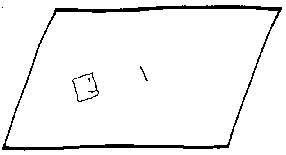I am here. I was not here yesterday. I was there last week. I might be here tomorrow. I might not. Where was I then? When was I there? I was there, then here's where I was way back when. How do I reconcile all of these "events"? If an event consists of a certain place at a certain time, how do I organize them? Space... time...Spacetime is, not surprisingly, the union of space and time. For our three-dimensional universe, each point in spacetime has four coordinates (three for position, one for time). Four dimensional geometries are diffucult to visualize, let alone comprehend. It is much more simple to imagine the spacetime of a two-dimensional universe. One such two-dimensional world is Edwin Abbott Abbott's creation, Flatland.Figure 1: A Square and Wife in Flatland

Flatlanders have two degrees of freedom: left/right and forward/backward. There is no up/down in their world. Therefore, in order to plot an event in Flatland, we need only three coordinates (two for position, one for time). It is possible to take a piece of Flatland space and a piece of Flatland time and incorporate them into a three-dimensional spacetime diagram:

Figure 2: Flatland Spacetime

In the diagram above, x and y represent space, and t represents time. Hence, to get an image of Flatland at a certain instant, one would look at a horizontal slice of the spacetime diagram. There are other ways to extract information from a spacetime diagram. For example, if one wanted to know all of the events that happened on any line on Flatland's surface, one would look at a planar slice that is perpendicular to the space of Flatland and passes through that line.

An alternative to slicing is projection. If one wanted to know whether A Square and Mrs. Square ever occupied the same space at different times, one could project their paths in spacetime (world lines) downward. If their shadows intersect, that means that they were at the same place at different times.

An important feature of spacetime is that it is relative. Figure 1 was rendered by a stationary observer outside of Flatland. A Square's version of this same chunk of spacetime would look like this:

Figure 3: Spacetime of Figure 2 According To A Square

In A Square's world, he is not moving--the world is moving around him. Of considerable notice here is that A Square's world line is shorter in Figure 3 than in Figure 2; and Mrs. Square's world line is longer in Figure 3 than in Figure 2. (For more on this, see time dilation). To get more of a "feel" for spacetime diagrams, one should examine the pictures below. Figure 4 is the piece of Flatland spacetime when/where A Sphere visits A Square. Notice that the sphere is static. This is because at any one instant, there was at most one slice of the sphere in Flatland. Therefore, the diagram only shows a series of two dimensional slices of the sphere. Figure 5 is a representation of the Flatlandian life (crunched down into several inches): A square is born, a line is born; they meet, have a baby square, and die.

Figure 4: A Sphere Visits Flatland

Figure 5: The Flatland Life Cycle

Spacetime diagrams make no implications about reality. It is very uncertain as to whether future spacetime is as static as past spacetime--if the past is static at all! There are also questions regarding the human role in spacetime. How does the human consciousness fit in? Some (Rudy Rucker) would argue that a Flatlander's perception of "now" can be represented by a plane moving through the spacetime diagram. More questions must be raised about this topic, and some of them are discussed in the accompanying pages.